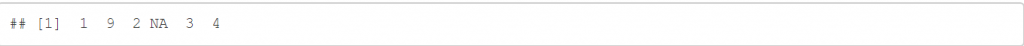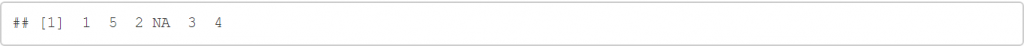#0

## [R語言]資料分析讀書心得06---使用dplyr做資料變換(select,mutate)

``````library(tidyverse)
library(nycflights13)
``````

``````glimpse(flights)
``````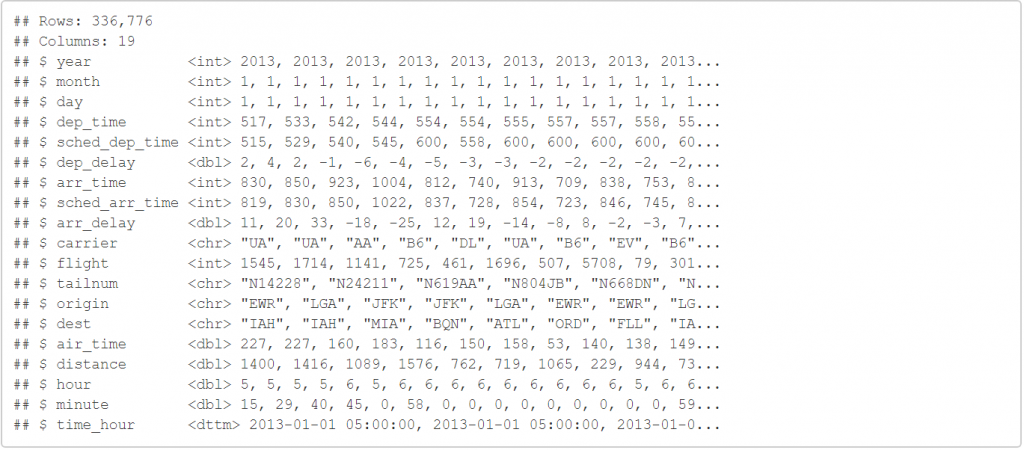``````select(flights,year,month,day)
``````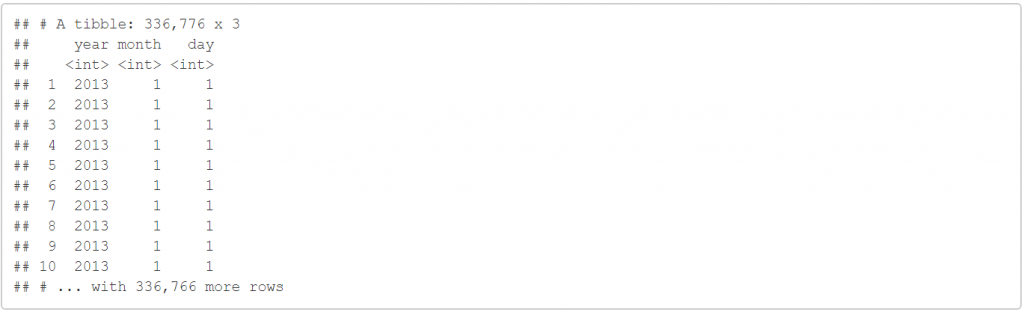``````select(flights,year:day)
``````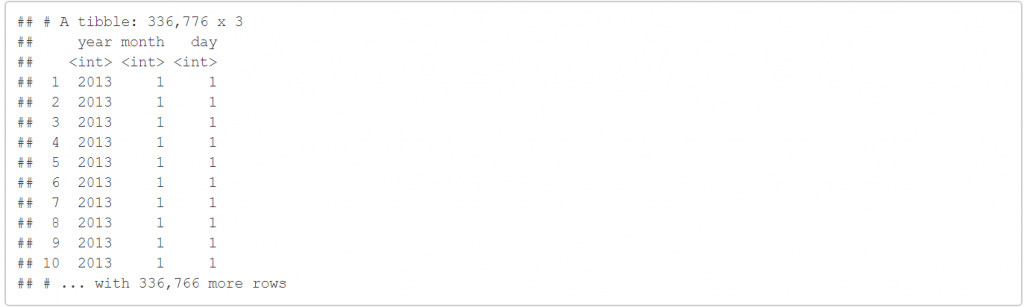``````select(flights,-(year:day))
``````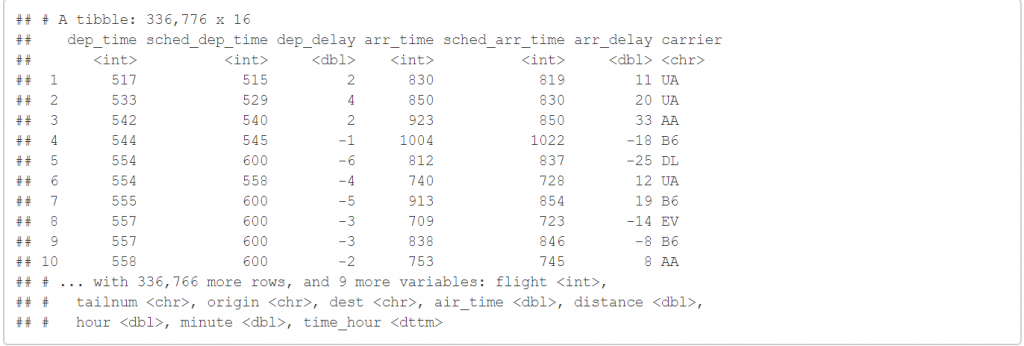``````select(flights,starts_with("dep"))
``````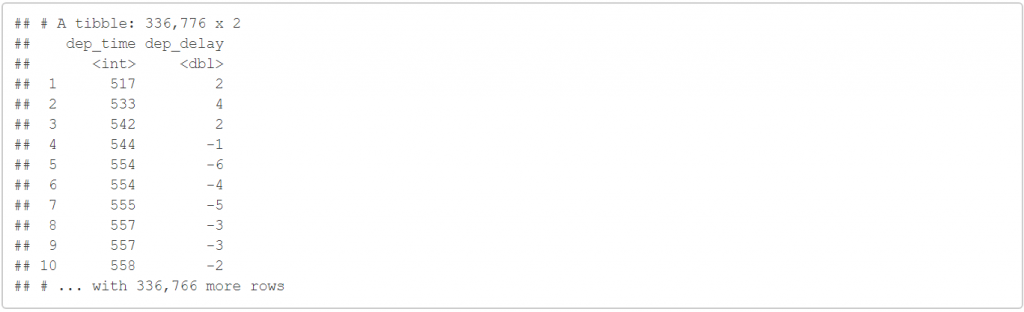starts_with ("dep") 會把開頭是"dep"的變數都留下來。

``````select(flights,ends_with("time"))
``````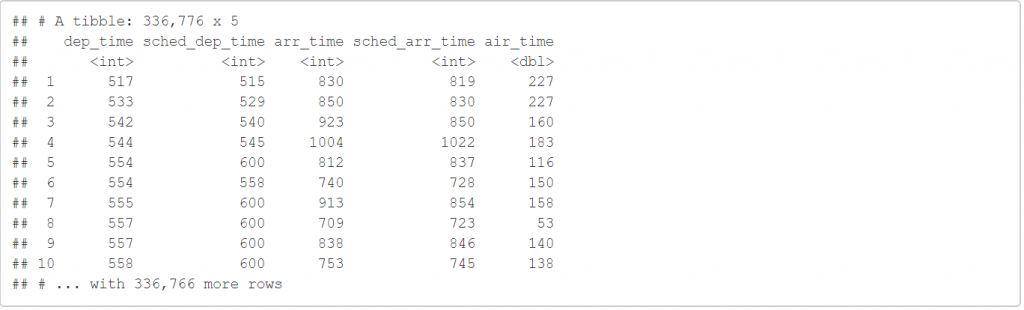ends_with("time") 會把結尾是"time"的變數都留下來。

``````select(flights,contains("rr"))
``````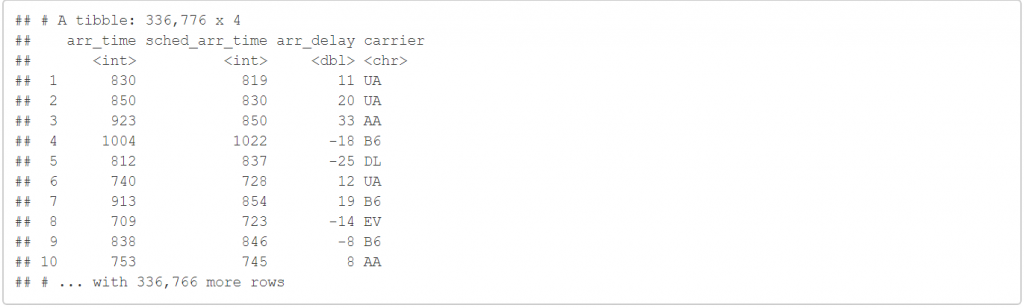contains("rr") 會把變數中含有"rr"字串的變數都留下來。

``````n_flights<-rename(flights,ABC=tailnum)
glimpse(n_flights)
``````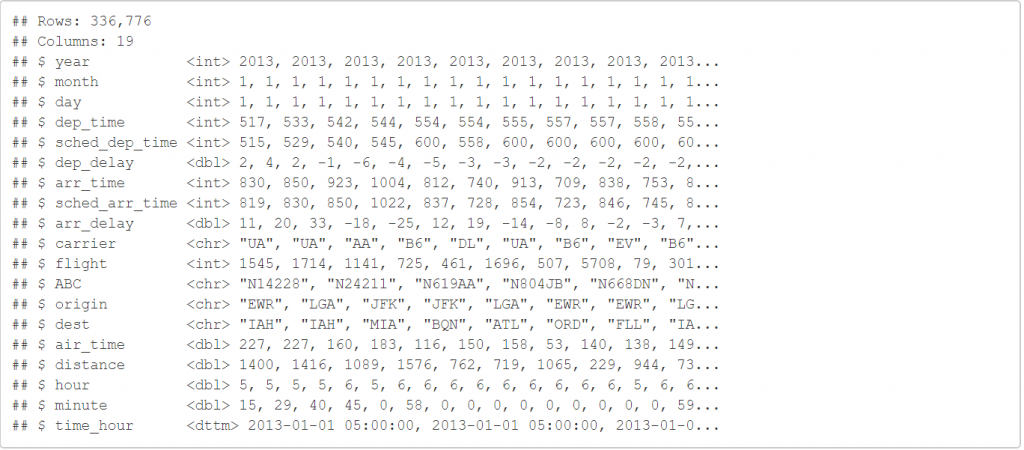``````select(flights,time_hour,air_time,everything())
``````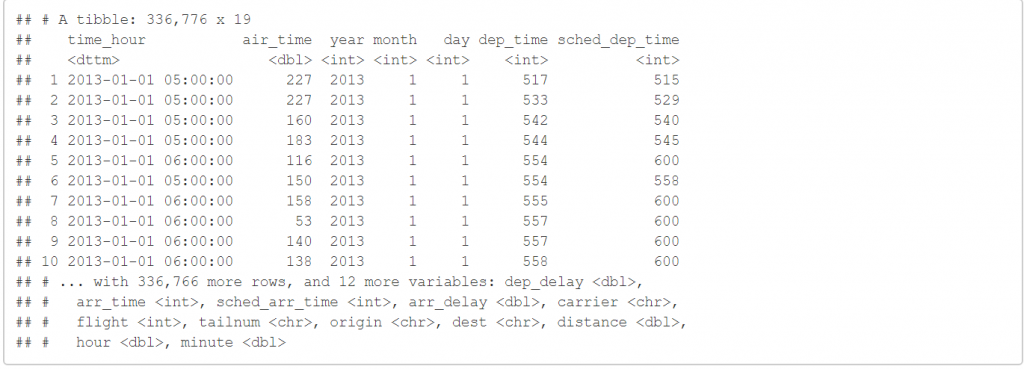``````mutate(flights,gain=arr_delay-dep_delay)
``````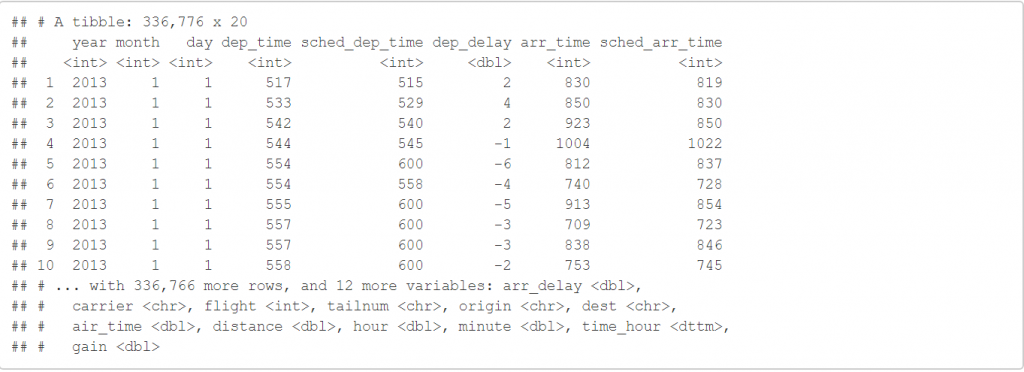``````transmute(flights,gain=arr_delay-dep_delay)
``````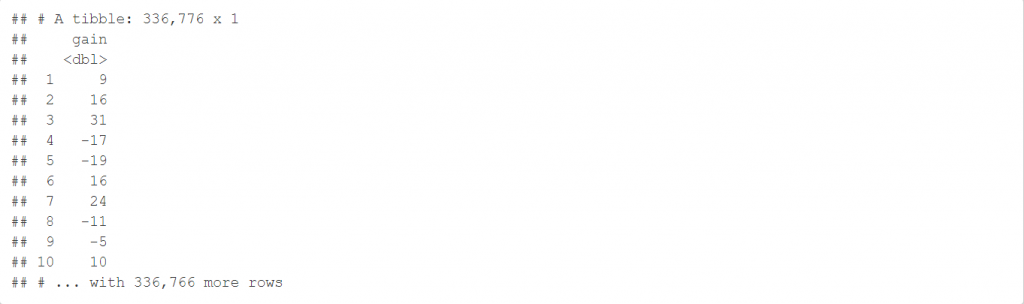``````transmute(flights,a=air_time/60)
``````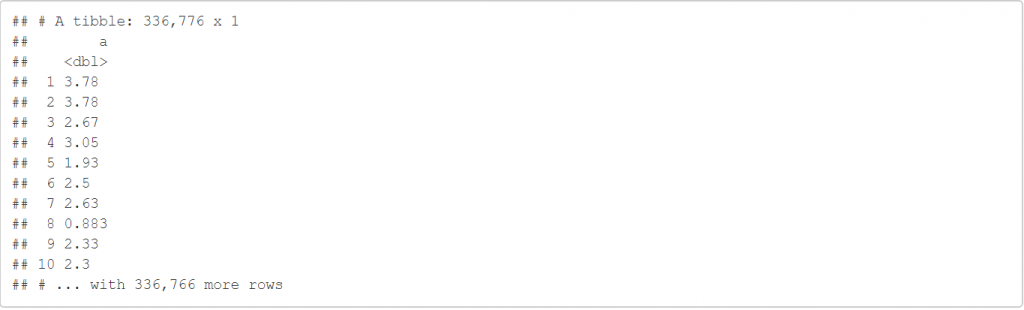``````transmute(flights,b=(sched_arr_time)/sum(sched_arr_time))
``````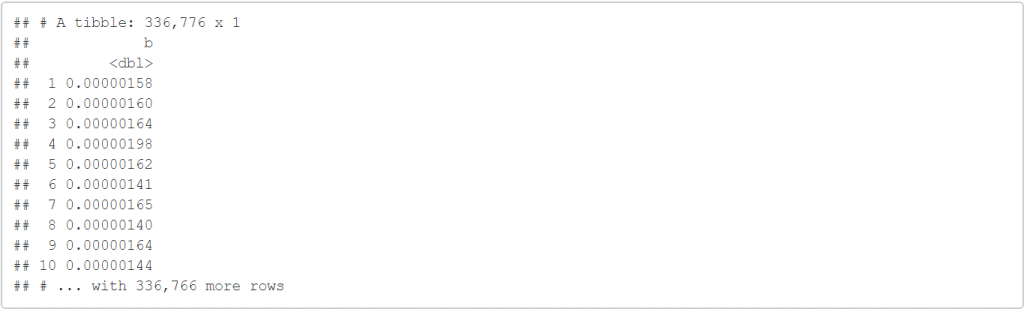``````transmute(flights,c=log(sched_arr_time))
``````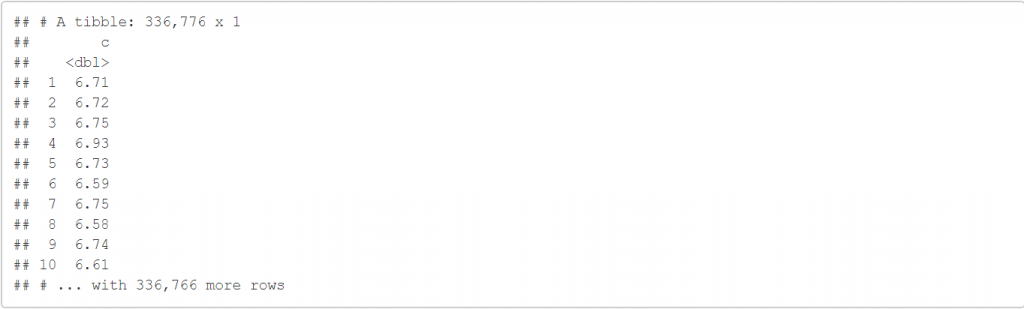``````(y<-c(1,9,2,NA,3,4))

min_rank(y)

``````